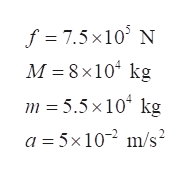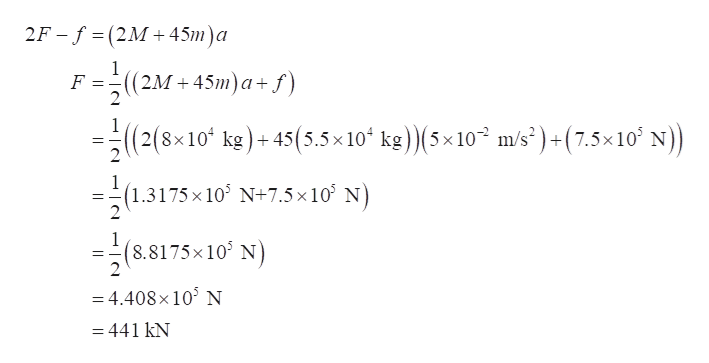A freight train consists of two 8.00x104 kg engines and 45 cars with average masses of 5.50x104 kg.  What force must each engine exert backward on the track to accelerate the train at a rate of 5.00x10-2 m/s2 if the force of friction is 7.50x105 N, assuming the engines exert identical forces?Group of answer choices882 kN441 kN221 kN110 kN

Question
A freight train consists of two 8.00x104 kg engines and 45 cars with average masses of 5.50x104 kg.  What force must each engine exert backward on the track to accelerate the train at a rate of 5.00x10-2 m/s2 if the force of friction is 7.50x105 N, assuming the engines exert identical forces?
Group of answer choices
882 kN
441 kN
221 kN
110 kN
Step 1

Let F be the force with which the engines pull the cars, f be the frictional force acting backwards on the track, m be the mass of the car, M be the mass of the engines, and a be the acceleration of freight train.

Write the values given in the question.help_outlineImage Transcriptionclosef = M 8 x10 kg 7.5x103 N m 5.5 x10 kg a 5x10 m/s fullscreen
Step 2

Draw the kinetic diagram of the motion of the freight train.

Step 3

Write the expression for Newton’s second...help_outlineImage Transcriptionclose2F - f (2M 45m)a 1 ((2M 45m)a+f F 2 1 (2(8x10 kg)+ 45(5.5x 10 kg)(5x10 m/s')+(7.5x10 N)) 1 (1.3175 x 10 N+7.5 x10 N 2 1 (8.8175x10' N 2 =4.408x 103 N = 441 kN fullscreen

Want to see the full answer?

See Solution

Want to see this answer and more?

Our solutions are written by experts, many with advanced degrees, and available 24/7

See Solution
Tagged in

Physics